﻿ 采煤机截割部行星传动轮系动力学分析

# 采煤机截割部行星传动轮系动力学分析Dynamic Analysis of the Planetary Gear Train in the Cutting Section of the Shearer

Abstract: In order to analyze the meshing transmission characteristics of planetary gear system of shearer cutting unit and the force condition of planet carrier, the rigid body dynamics simulation analysis of planetary transmission system is carried out by ADAMS, and transient analysis is done by com-bining ANSYS. The simulation results show that the levels of the meshing forces between the plan-etary gear and sun gear are similar to those of theoretical results. The meshing forces in X and Y direction between sun gear and planetary gear change periodically with a phase difference of 90 DEG. The stresses at the spline join and at the root of the side panel of the secondary planetary frame are larger, which is the dangerous positions. The simulation results verify the gear trans-mission characteristics of the planetary gear train, and provide the basis for the study of planetary carrier to get the dangerous position of the two-stage planetary frame structure, providing a ref-erence for the design and research of planetary frame.

1. 引言

2. 行星轮系建模与刚体动力学仿真

2.1. 传动系统刚体模型建立

2.2. 添加约束与接触

1) 添加约束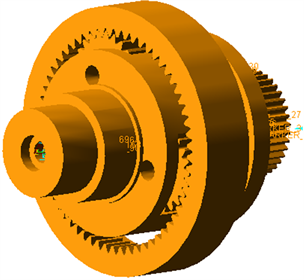Figure 1. Rigid body dynamics simulation model

2) 添加接触

$impact\left(x,\stackrel{\cdot }{x,{x}_{1},K,e,c,d}\right)=\left\{\begin{array}{c}\mathrm{max}\left(0,K{\left({x}_{1}-x\right)}^{e}-step\left(x,{x}_{1}-d,c,{x}_{1},0\right)\stackrel{\cdot }{x}\right)\\ 0\end{array}\begin{array}{c}x<{x}_{1}\\ x\ge {x}_{1}\end{array}$ (1)

$P=K\cdot {\delta }^{3/2}$ (2)

$\left\{\begin{array}{l}K=\frac{4}{3}{R}^{1/2}E\\ \frac{1}{R}=\frac{1}{{R}_{1}}±\frac{1}{{R}_{2}}\\ \frac{1}{E}=\frac{\left(1-{\mu }_{1}^{2}\right)}{{E}_{1}}+\frac{\left(1-{\mu }_{2}^{2}\right)}{{E}_{2}}\end{array}$ (3)

2.3. 添加驱动和负载

3. 刚体动力学仿真结果及分析

3.1. 仿真模型验证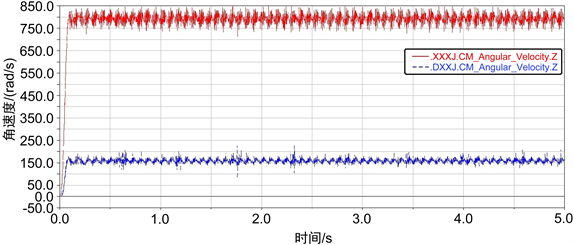Figure 2. Angular velocity of the planetary frame

3.2. 行星轮系齿轮啮合力分析

$\begin{array}{l}T=9549\frac{P}{n}\\ {F}_{sr}=\frac{2000T}{{n}_{p}\cdot {d}_{s}}\end{array}$ (4)

$\left\{\begin{array}{l}{F}_{r1s1x}={F}_{r1s1}\mathrm{sin}\left(\omega t+\alpha \right)\\ {F}_{r1s1y}={F}_{r1s1}\mathrm{cos}\left(\omega t+\alpha \right)\end{array}$ (5)Figure 3. Meshing force of gear

4. 二级行星架瞬态动力学分析

4.1. 瞬态动力学模型建立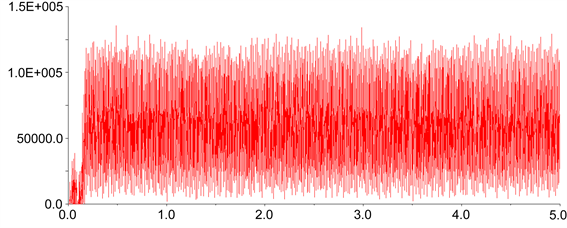(a) 一级行星齿轮与太阳轮啮合力曲线(b) 一级行星齿轮与太阳轮X向啮合力曲线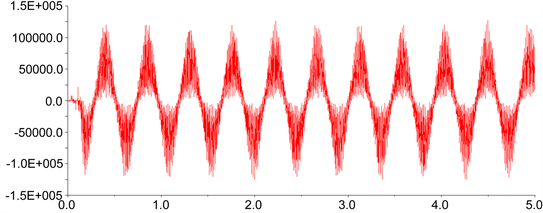(c) 一级行星齿轮与太阳轮Y向啮合力曲线

Figure 4. First planetary gear and solar wheel meshing force curve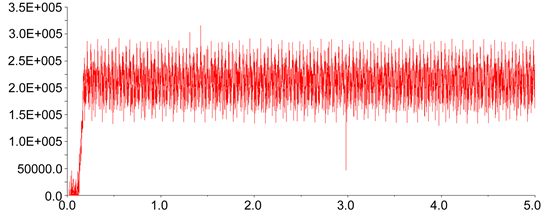(a) 二级行星齿轮与太阳轮啮合力曲线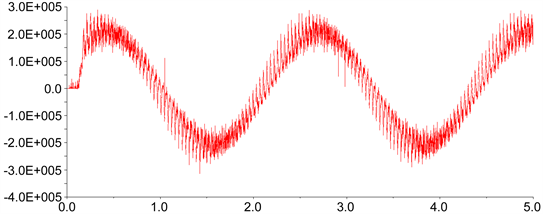(b) 二级行星齿轮与太阳轮X向啮合力曲线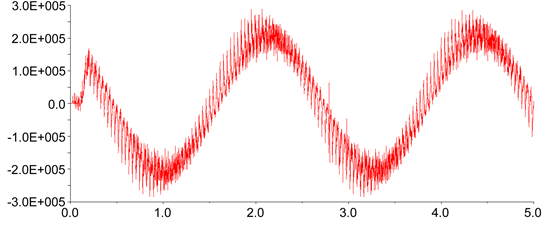(c) 二级行星齿轮与太阳轮Y向啮合力曲线

Figure 5. The meshing force curve of the two stage planetary gear and the sun wheel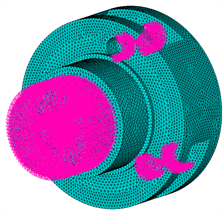(a)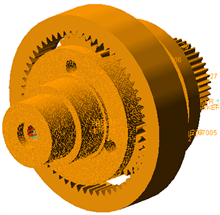(b)

Figure 6. Rigid flexible coupling model of two stage planetary frame

4.2. 对刚柔耦合模型添加驱动和负载

4.3. 仿真结果分析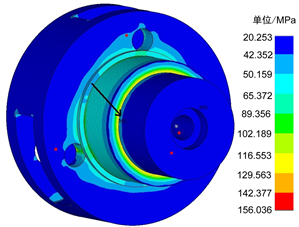(a)等效应力云图1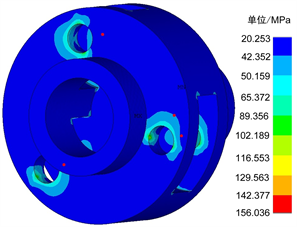(b) 等效应力云图2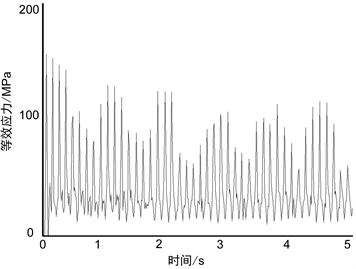(c) Node17624节点应力时间历程曲线

Figure 8. Stress cloud and time history curve of the two stage planetary frame

5. 结论

NOTES

*通讯作者。

 赵丽娟, 王乘云. 采煤机截割部建模与动力学仿真研究[J]. 工程设计学报, 2010, 17(2): 119-123.

 徐健博. 采煤机截割部行星架疲劳寿命分析[J]. 煤矿机电, 2017(4): 72-75.

 郭生龙. 电牵引采煤机截割部摇臂瞬态动力响应分析[J]. 煤矿机械, 2013, 34(1): 103-104.

 赵丽娟, 李佳, 田震, 车小贺. 新型薄煤层采煤机截割部建模与仿真研究[J]. 机械传动, 2013, 37(1): 47-50.

 毛君, 刘晓宁, 陈洪月, 等. 采煤机截割部传动系统刚柔耦合动力学仿真分析[J]. 机械强度, 2017, 39(5): 1138-1144.

 周游, 李国顺, 唐进元. 截齿截割煤岩的LS-DYNA仿真[J]. 工程设计学报, 2011, 18(2): 103-108.

 刘楷安, 李秋菊, 姜学寿. 基于刚柔耦合的采煤机摇臂动态特性仿真研究[J]. 煤矿机械, 2010, 31(11): 47-49.

 赵丽娟, 乔美娜, 兰金宝. 新型采煤机截割部行星轮系强度分析[J]. 机械传动, 2015, 39(2): 107-110+123.

 赵丽娟, 孙影. 采煤机截割部的刚柔耦合动力学分析[J]. 机械传动, 2011, 35(6): 68-71.

 张克斌, 杨兆建, 王义亮. 电牵引采煤机截割部动力学分析[J]. 煤矿机械, 2012, 33(8): 88-90.

 吴卫东, 安兴伟. 基于ANSYS的采煤机摇臂的有限元分析[J]. 煤矿机械, 2009, 30(3): 77-79.

 郭会珍. 滚筒式采煤机截割部动力学特性研究[D]: [硕士学位论文]. 徐州: 中国矿业大学, 2014.

Top